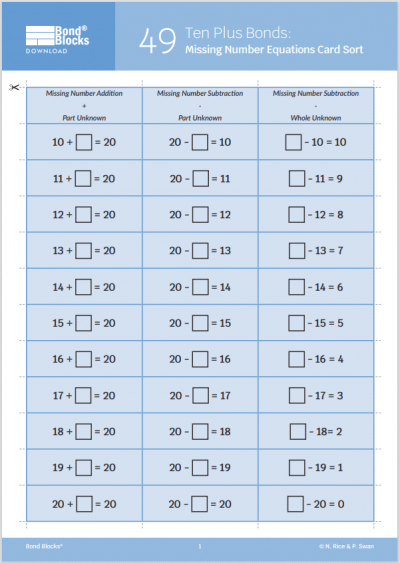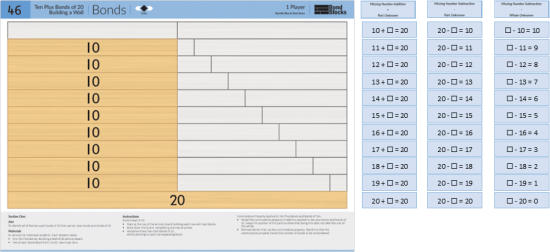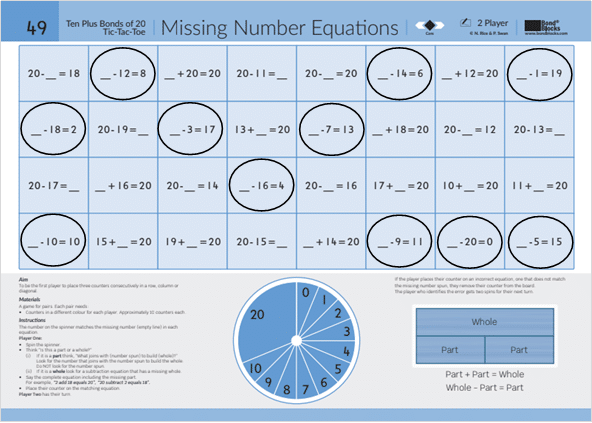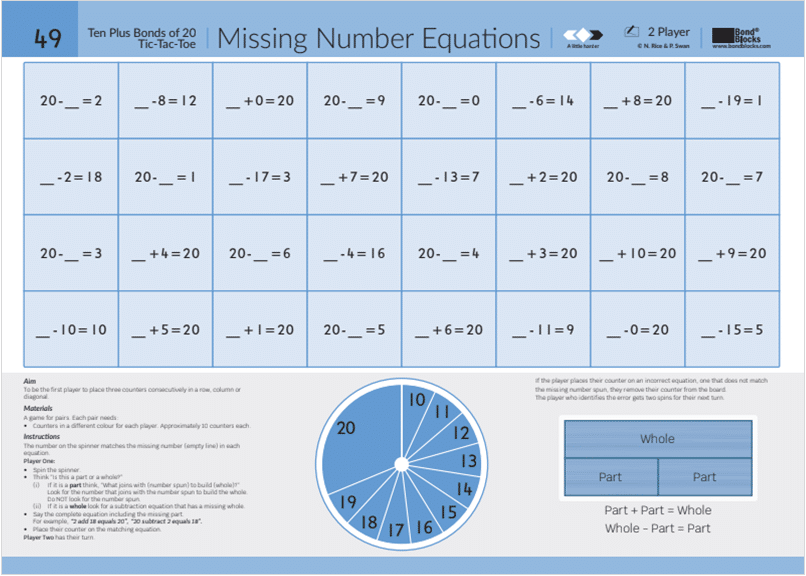## 49) Missing Number Equations

Ten Plus Bonds: Tic-Tac-Toe### Mathematics

Develop the concept of using part-part-whole, to calculate an unknown part or whole, in addition and subtraction equations, for ten plus bonds of 20.

This is a complex concept that has been highly scaffolded in previous Missing Number Activities.

• First, students need to identify if the unknown number could be in the position of a part or a whole.
• Unknown parts can be calculated by either adding on or subtracting. For example, 20-?=13 can be solved by
(i) adding on to the known part to reach the whole, 13+?=20 or
(ii) making a subtraction equation where the unknown part is in the answer position, 20-13=?
• Unknown wholes are calculated by adding the known parts.

The missing number equations in Activity 49 are placed in random order. This is more difficult than the previous Missing Number Core Activities, where equations were grouped together in rows of similar type.

### Language

• subtraction as ‘subtract’. Read aloud as “(Whole) subtract (part) equals (part)”.
• equals
• part-part-whole
• missing part solved by thinking, “What joins with (number spun) to build 20?”
• missing whole solved by thinking, “Look for a subtraction equation what has a missing whole.”
• The activity board uses the word “missing”. The word “unknown” is introduced when the Missing Number Sorting Cards are used.## Differentiation

### A little easier

##### Card sort

Click to download the  Each card is one of the equations from the Missing Number Equations game board. Instruct students to:

• Cut out each card.
• Mix the cards.
• Sort the cards into three groups using the diagram heading cards:
• subtraction: one part unknown
• subtraction: whole unknownAfter sorting the cards. Instruct students to:

• Build a two-part bond wall for 20 by placing Bond Blocks on Activity Board 46.
• Start with the addition cards in a jumbled pile. Sort the cards, placing them next to the matching row of Bond Blocks.
• Use a pencil to fill in the missing number on each addition card.
• Repeat for the subtraction cards with an unknown part.
• Repeat for the subtraction cards with an unknown whole.
• Discuss strategies for calculating the unknown, including the importance of first identifying if it is a missing part or whol##### Identify missing whole equations
• Place the activity board in a write and wipe sleeve. Circle all the subtraction equations where the missing number is in the whole position.
• Either play or solve each missing number equation. Record this missing number on the write and wipe sleeve using a dry erase marker.### A little harder

##### Missing number equations tic-tac-toe

Play Ten Plus Bonds of 20: Missing Number Equations Tic-Tac-Toe a little harder. This game board is harder compared to the core game board because the number spun is a two-digit number.### Progression

In the next section of activities students apply Ten Plus Bonds to develop the addition and subtraction strategy of bridging ten. Go to

##### Activity 50

Ten Plus Bonds: Bridging Ten Addition, Strategy 9+